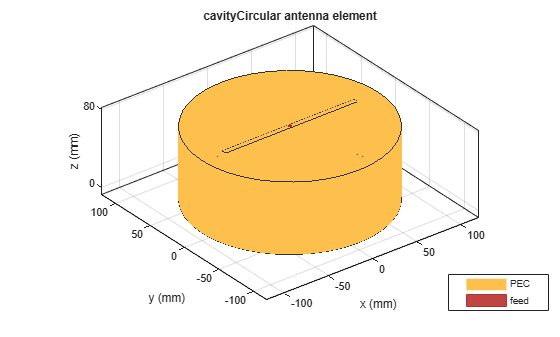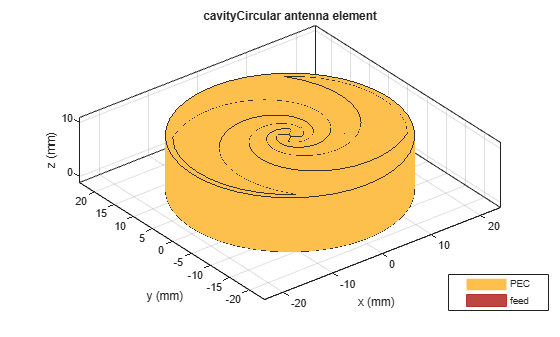Documentation

### This is machine translation

Mouseover text to see original. Click the button below to return to the English version of the page.

# cavityCircular

Create circular cavity-backed antenna

## Description

Use the `circularCavity` object to create a circular cavity-backed antenna. By default, the exciter used is a dipole. The dimensions are chosen for an operating frequency of 1 GHz.## Creation

### Syntax

``circularcavity = cavityCircular``
``circularcavity = cavityCircular(Name,Value)``

### Description

example

````circularcavity = cavityCircular` creates a circular cavity-backed antenna. ```

example

````circularcavity = cavityCircular(Name,Value)` sets properties using one or more name-value pairs. For example, `circularcavity = cavityCircular('Radius',0.2)` creates a circular cavity of radius 0.2 m. Enclose each property name in quotes.```

## Properties

expand all

Antenna type used as an exciter, specified as an object. Except for reflector and cavity antenna elements, you can use any of the single elements in the Antenna Toolbox™ as an exciter.

Example: `'Exciter',monopole`

Example: ```circularcavity.Exciter = monopole```

Data Types: `char` | `string`

Radius of cavity, specified as a scalar in meters.

Example: `'Radius',0.2`

Example: `circularcavity.Radius = 0.2`

Data Types: `double`

Cavity height along z-axis, specified as a scalar in meters.

Example: `'Height',0.001`

Example: `circularcavity.Height = 0.001`

Data Types: `double`

Distance between the exciter and the base of the cavity, specified a scalar in meters.

Example: `'Spacing',7.5e-2`

Example: `circularcavity.Spacing = 7.5e-2`

Data Types: `double`

Type of dielectric material used as a substrate, specified as a object. For more information see, `dielectric`. For more information on dielectric substrate meshing, see Meshing.

### Note

The substrate dimensions must be equal to the groundplane dimensions.

Example: ```d = dielectric('FR4'); 'Substrate',d```

Example: ```d = dielectric('FR4'); circularcavity.Substrate = d```

Create probe feed from backing structure to exciter, specified as `0` or `1` or a positive scalar. By default, probe feed is not enabled.

Example: `'EnableProbeFeed',1`

Example: ```circularcavity.EnableProbeFeed = 1```

Data Types: `double` | `logical`

Lumped elements added to the antenna feed, specified as a lumped element object handle. For more information, see `lumpedElement`.

Example: `'Load',lumpedelement`. `lumpedelement` is the object handle for the load created using `lumpedElement`.

Example: ```circularcavity.Load = lumpedElement('Impedance',75)```

Tilt angle of the antenna, specified as a scalar or vector with each element unit in degrees. For more information, see Rotate Antenna and Arrays.

Example: `'Tilt',90`

Example: `'Tilt',[90 90]``'TiltAxis',[0 1 0;0 1 1]` tilts the antenna at 90 degree about two three-element vector points in space.

Data Types: `double`

Tilt axis of the antenna, specified as:

• Three-element vectors of Cartesian coordinates in meters. In this case, each vector starts at the origin and lies along the specified points on the X-, Y-, and Z- axes.

• Two points in space, each specified as three-element vectors of Cartesian coordinates. In this case, the antenna rotates around the line joining the two points in space.

• A string input describing simple rotations around one of the principal axes, 'X', 'Y', or 'Z'.

Example: `'TiltAxis',[0 1 0]`

Example: `'TiltAxis',[0 0 0;0 1 0]`

Example: `ant.TiltAxis = 'Z'`

## Object Functions

 `show` Display antenna or array structure; Display shape as filled patch `axialRatio` Axial ratio of antenna `beamwidth` Beamwidth of antenna `charge` Charge distribution on metal or dielectric antenna or array surface `current` Current distribution on metal or dielectric antenna or array surface `design` Design prototype antenna or arrays for resonance at specified frequency `EHfields` Electric and magnetic fields of antennas; Embedded electric and magnetic fields of antenna element in arrays `impedance` Input impedance of antenna; scan impedance of array `mesh` Mesh properties of metal or dielectric antenna or array structure `meshconfig` Change mesh mode of antenna structure `pattern` Radiation pattern and phase of antenna or array; Embedded pattern of antenna element in array `patternAzimuth` Azimuth pattern of antenna or array `patternElevation` Elevation pattern of antenna or array `returnLoss` Return loss of antenna; scan return loss of array `sparameters` S-parameter object `vswr` Voltage standing wave ratio of antenna

## Examples

collapse all

Create and view a default circular cavity-backed antenna.

`a = cavityCircular`
```a = cavityCircular with properties: Exciter: [1x1 dipole] Substrate: [1x1 dielectric] Radius: 0.1000 Height: 0.0750 Spacing: 0.0750 EnableProbeFeed: 0 Tilt: 0 TiltAxis: [1 0 0] Load: [1x1 lumpedElement] ```
`show(a)`Create and view an equiangular spiral backed by a circular cavity. The cavity dimensions are:

Height = 0.01 m

Spacing = 0.01 m

``` ant = cavityCircular('Exciter',spiralEquiangular,'Radius',0.02, ... 'Height',0.01,'Spacing', 0.01); show(ant) ```